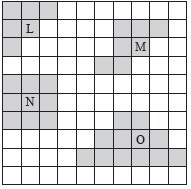# User ForumSubject :IMO    Class : Class 5

Select a pair from the given shapes which has the same perimeter.AL and M
BM and O
CL and N
DN and O

Class : Class 1

(C); Count the outer edges of each shape, consider each segment as one unit.
Perimeter of L = 12, Perimeter of M = 14, Perimeter of N = 12, Perimeter of O = 18
L and N has same perimeter

Class : Class 5